Approximants derived by expanding a function as a ratio of two Power Series and determining both the Numerator and Denominator Coefficients. Padé approximations are usually superior to Taylor Expansions when functions contain Poles, because the use of Rational Functions allows them to be well-represented.

The Padé approximant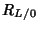corresponds to the Maclaurin Series. When it exists, thePadé approximant to any Power Series(1)

is unique. If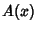is a Transcendental Function, then the terms are given by the Taylor Series about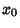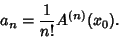(2)

The Coefficients are found by setting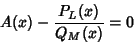(3)

and equating Coefficients.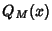can be multiplied by an arbitrary constant which will rescale the other Coefficients, so an addition constraint can be applied. The conventional normalization is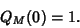(4)

Expanding (3) gives(5)(6)

These give the set of equations(7)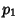(8)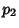(9)(10)(11)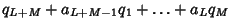(12)

wherefor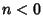andfor. Solving these directly gives(13)

where sums are replaced by a zero if the lower index exceeds the upper. Alternate forms arefor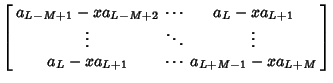(14)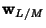(15)

and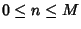.

The first few Padé approximants forare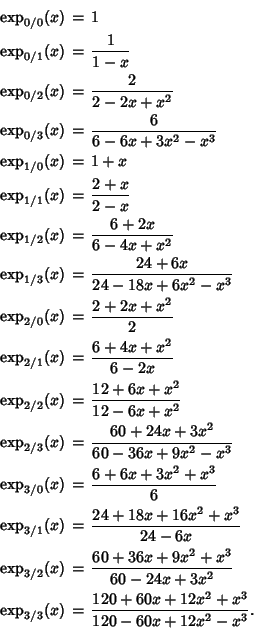Two-term identities include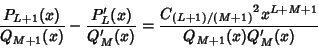(16)(17)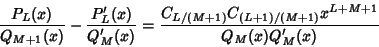(18)(19)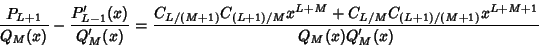(20)(21)

whereis the C-Determinant. Three-term identities can be derived using the Frobenius Triangle Identities (Baker 1975, p. 32).

A five-term identity is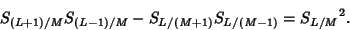(22)

Cross ratio identities include(23)(24)(25)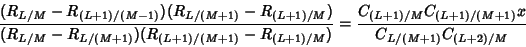(26)(27)

References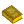Padé Approximants

Baker, G. A. Jr. The Theory and Application of The Pade Approximant Method.'' In Advances in Theoretical Physics, Vol. 1 (Ed. K. A. Brueckner). New York: Academic Press, pp. 1-58, 1965.

Baker, G. A. Jr. Essentials of Padé Approximants in Theoretical Physics. New York: Academic Press, pp. 27-38, 1975.

Baker, G. A. Jr. and Graves-Morris, P. Padé Approximants. New York: Cambridge University Press, 1996.

Press, W. H.; Flannery, B. P.; Teukolsky, S. A.; and Vetterling, W. T. Padé Approximants.'' §5.12 in Numerical Recipes in FORTRAN: The Art of Scientific Computing, 2nd ed. Cambridge, England: Cambridge University Press, pp. 194-197, 1992.